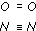Water for life

Start this free course now. Just create an account and sign in. Enrol and complete the course for a free statement of participation or digital badge if available.

Free course

# 6.3 Chemical formulas

By using symbols, elements can be represented much more conveniently and much more briefly. This method of using symbols can be extended to compounds. You will now look further into this idea using a very familiar compound: water. Recall which atoms there are in a water molecule.

## Question 24

What symbols would you use to represent the water molecule?

Since the water molecule has two hydrogen atoms and one oxygen atom, you might have written down HHO, HOH or OHH.

It is conventional to add up all the atoms of one type in a molecule, so it is written H2O where the subscript 2 after the H indicates that there are two hydrogen atoms and the absence of a subscript after O indicates that there is only one oxygen atom. Such a representation is known as a chemical formula. Arguably, you should write H2O1 but for convenience and simplicity the subscript 1 is always omitted. Unfortunately, there is no obvious rule to indicate which element should be written down first in a chemical formula. Do you write H2O or OH2? You probably know the answer, of course; from saying 'aitch-two-oh': you would write H2O. The reason is, essentially, a matter of convention: that's the way chemists do it. At first, you may find this system of writing formulas slightly awkward. Concentrate on remembering that the subscript refers to the symbol that directly precedes it. (There are two possible plurals for formula: we will use 'formulas' but you may also see 'formulae' in some textbooks.)

## Question 25

Write down the chemical formulas for carbon dioxide and methane. You may need to refer back to previous sections to remind yourself which atoms are present and in what proportions.

The chemical formula for carbon dioxide is CO2 (pronounced 'see-oh-two') and for methane is CH4 (pronounced 'see-aitch-four').

The chemical formula of a covalent compound shows the number of each type of atom in one molecule of the compound. It is written using the symbols for the elements.

Having examined the naming of elements, their symbols and the formulas of compounds, what about the names of compounds? As with elements, the everyday names of some compounds have their origins in history. For example, words that sound like 'water' have been used for this liquid for thousands of years. The old English term was 'waeter', in old Saxon 'watar', in old German 'wazzar' and ancient Greek 'hudor'. For common compounds these old names linger on: water, ammonia, salt and alcohol are just some examples. However, there are millions of different compounds - if they all had common names you would never be able to remember them. A more simple, logical naming system is needed, which can be applied to any compound, so that everyone can understand which compound is being talked about.

The scientific name reflects the elements found in the compound. Where a compound contains just two elements, the name of the second element is usually modified slightly so that it ends in the letters '-ide' (pronounced as in 'side'). Thus, the compound HCl (pronounced 'aitch-see-ell') is hydrogen chloride not hydrogen chlorine. Similarly, CO2 is carbon dioxide not carbon dioxygen. The 'di-' prefix indicates that there are two oxygen atoms and the '-ide' ending confirms that only two elements are involved in it.

Now try Questions 15 to 18, referring to Table 4 and Table 5 where necessary.

## Question 15

Using chemical symbols to represent the atoms and the information in Section 5, write structures for (a) a molecule of oxygen and (b) a molecule of nitrogen. Draw covalent bonds as straight lines and not joined hooks. For example, the structure for water would be written as H—O—H.You may have gone through the stage of drawing linked hooks to get to these molecular structures showing double and triple covalent bonds, respectively. (Note: a triple covalent bond is the maximum allowed between two atoms; quadruple bonds between two atoms do not exist.)

## Question 16

Which elements make up the following compounds? What is the ratio of the constituent atoms within each molecule?

(a) Ammonia, NH3 (pronounced 'en-aitch-three')

(b) Hydrogen sulfide, H2S (pronounced 'aitch-two-ess').

(a) Nitrogen and hydrogen: the atoms are in the ratio of 1 : 3 within the molecule of NH3 (called ammonia).

(b) Hydrogen and sulfur: the atoms are in the ratio of 2 : 1, respectively, within the molecule of H2S (called hydrogen sulfide).

## Question 17

Write the chemical symbols for the elements that make up the following compounds.

(a) Hydrogen bromide

(b) Silicon dioxide

(c) Nitrogen trichloride ('tri-' is the prefix for three atoms).

The chemical symbol for: (a) hydrogen is H, bromine is Br; (b) silicon is Si, oxygen is O; (c) nitrogen is N, chlorine is Cl.

## Question 18

Given that bromine, silicon and nitrogen normally form one, four and three covalent bonds respectively, and drawing on knowledge you already have about hydrogen and oxygen, write down the chemical formulas of the compounds named in Question 17. (You can write down the formulas from the names alone by using 'di', 'tri' and 'ide'. If you do this, use your understanding of covalent bonding to check your answers.)

The chemical symbol for hydrogen bromide is HBr, silicon dioxide is SiO2, nitrogen trichloride is NCl3.

(This ties in with their names, with the meaning of 'di-' and 'tri-', and with the idea of a 'preferred' number of covalent bonds.)

S154_1